Function Repository Resource:

# ArithmeticProgression

Produce an arithmetic progression with a specified difference

Contributed by: Pedro Cabral
 ResourceFunction["ArithmeticProgression"][n,d] produces an arithmetic progression of n terms starting at d and incrementing by d. ResourceFunction["ArithmeticProgression"][n,d,a] produces an arithmetic progression starting term at a rather than d.

## Examples

### Basic Examples (3)

Compute the first 10 terms of an arithmetic progression with a common difference of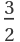:

 In:=Out=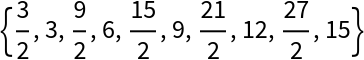Perform a ListLinePlot of different arithmetic progressions:

 In:=Out=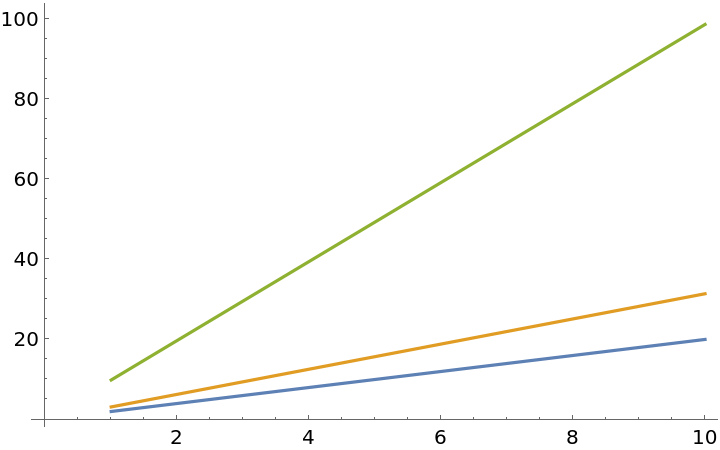Get 30 terms with a common difference of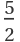, starting at 20:

 In:=Out=### Scope (1)

ArithmeticProgression can handle complex numbers, symbols and constants:

 In:=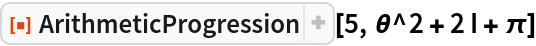Out=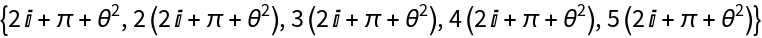### Properties and Relations (3)

An arithmetic progression of common difference 1 is the same as a Range:

 In:=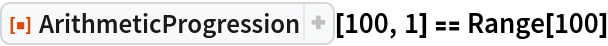Out=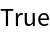The Mean of an arithmetic progression is the same as its Median:

 In:=Out=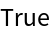The sum of all terms is the half the length of the progression times the first term plus the last term:

 In:=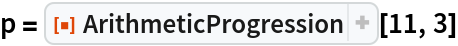Out=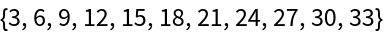In:=Out=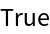Pedro Cabral

## Version History

• 1.0.0 – 09 September 2020